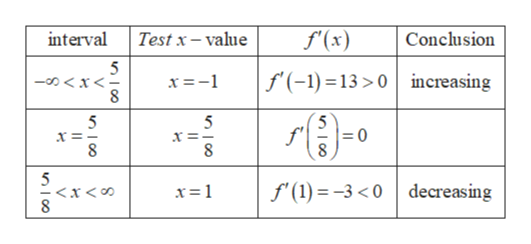# Find all relative extrema of the function. (If an answer does not exist, enter DNE.)f(x) = −4x2 + 5x + 8relative maximum    (x, y) =        relative minimum    (x, y) =

Question
90 views

Find all relative extrema of the function. (If an answer does not exist, enter DNE.)

f(x) = −4x2 + 5x + 8
relative maximum     (x, y)  =

relative minimum     (x, y)  =
check_circle

Step 1

Finding f’(x)

To find the relative extremum points of the function, we must use first derivative of the function,

Step 2

Finding all the critical points and all points where f is undefined.

The critical points of a function f are x-values within the domain of f for which f’(x) = 0 or

Where the function is defined and its derivative is zero and undefined.

So, critical points are:

Step 3

Analysing intervals of increase or decrease:

T...help_outlineImage Transcriptioncloseinterval f(x) Test x-value Conclusion 5 -ox<= 8 f(-1)13>0increasing x =-1 5 8 8 5 x< 8 f (1)-3<0 decreasing nco fullscreen

### Want to see the full answer?

See Solution

#### Want to see this answer and more?

Solutions are written by subject experts who are available 24/7. Questions are typically answered within 1 hour.*

See Solution
*Response times may vary by subject and question.
Tagged in

### Calculus